## Figures index

#### C. I. Nwoye, P. C. Nwosu, E. C. Chinwuko, S. O. Nwakpa, I. E. Nwosu, N. E. Idenyi

American Journal of Mining and Metallurgy. 2014, 2(3), 57-63 doi:10.12691/ajmm-2-3-4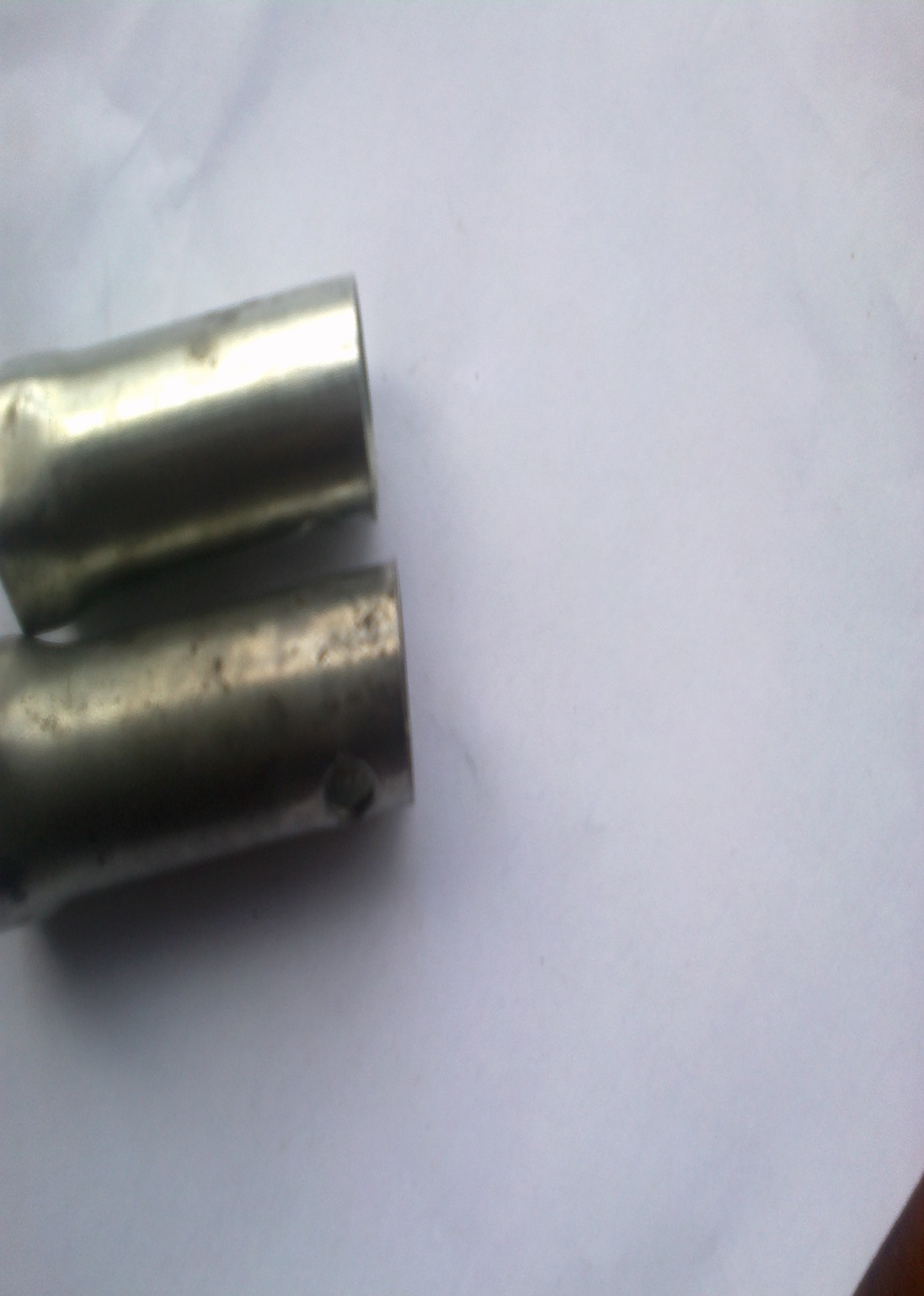• Figure 1. As-cast Al-Mn alloy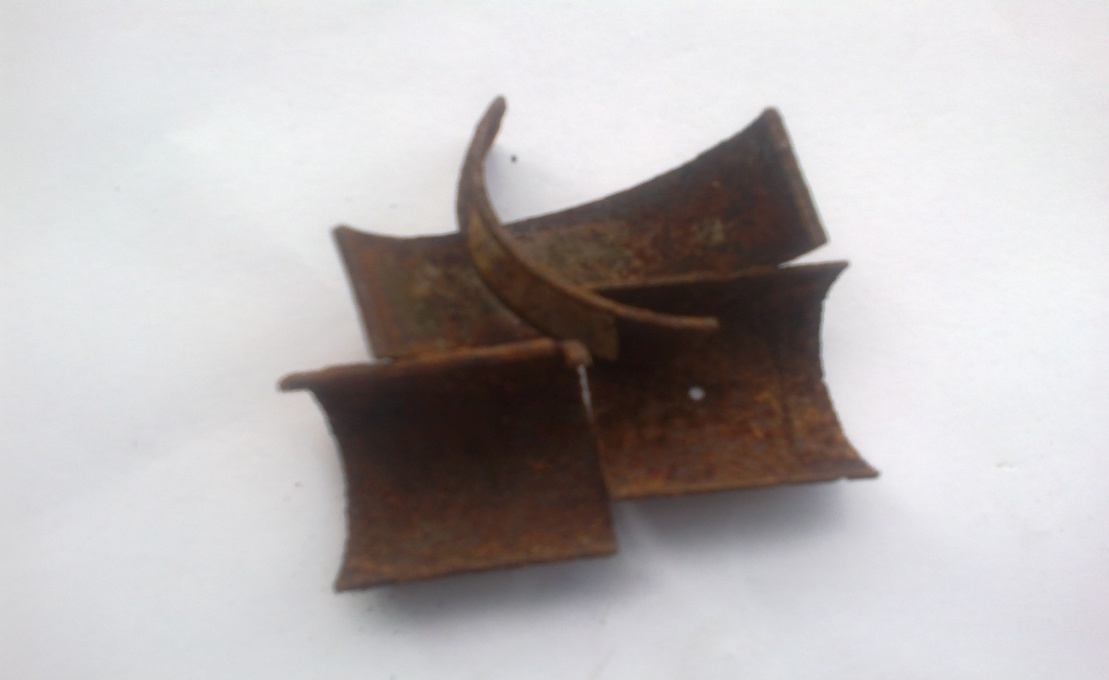• Figure 2. Corroded pieces of Al-Mn alloy cut and exposed to sea water environment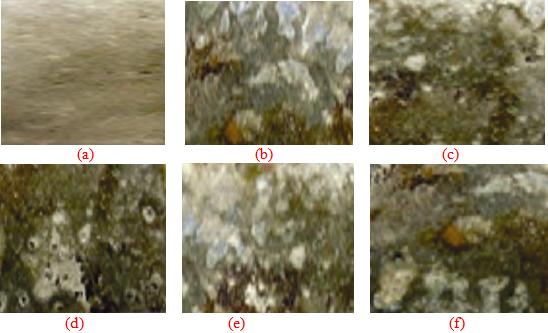• Figure 3. Surface structure of Al-Mn alloy (a) control (b), (c) (d), (e) and (f) for as-cast manganese contents: 2, 2.5, 2.8, 3 and 4 wt % respectively. (x200)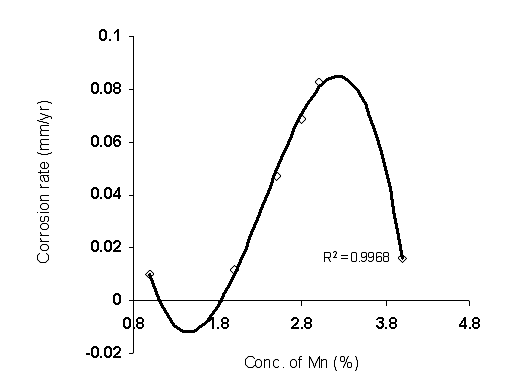• Figure 4. Coefficient of determination between Al-Mn alloy corrosion rate and as-cast manganese content as obtained from the experiment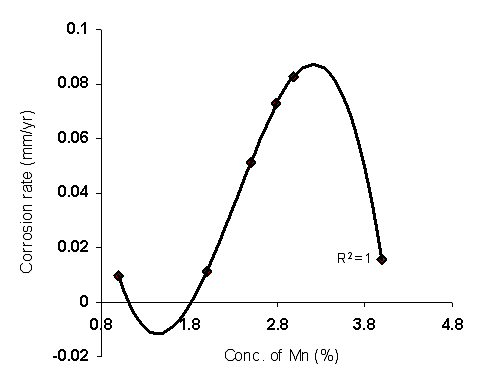• Figure 5. Coefficient of determination between Al-alloy Mn corrosion rate and as-cast manganese content as predicted by derived model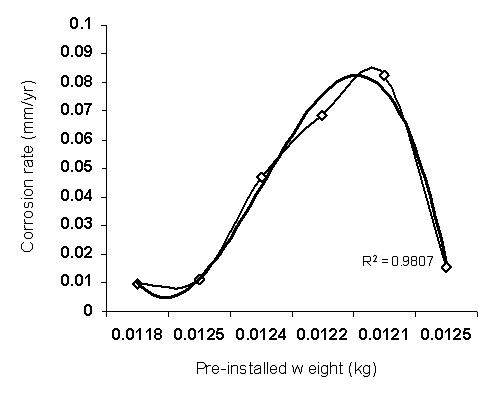• Figure 6. Coefficient of determination between Al-Mn alloy corrosion rate and its pre-installed weight as obtained from the experiment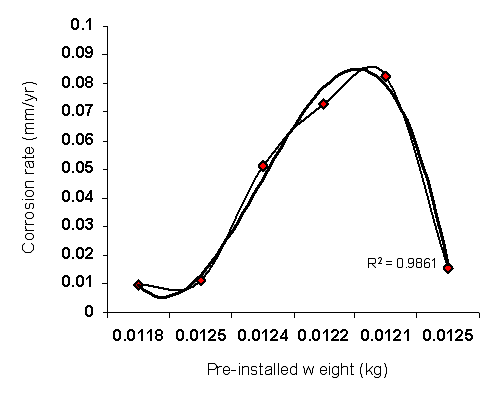• Figure 7. Coefficient of determination between Al-Mn alloy corrosion rate and its pre-installed weight as obtained from the experiment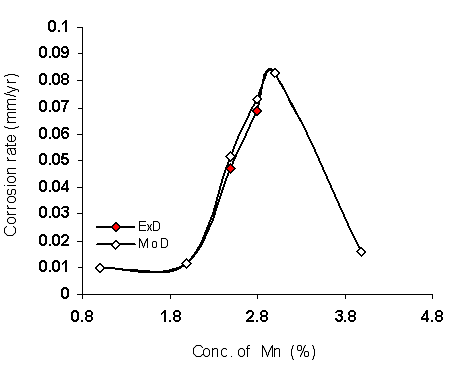• Figure 8. Comparison of the Al-Mn alloy corrosion rate (relative to as-cast manganese content) as obtained from experiment and derived model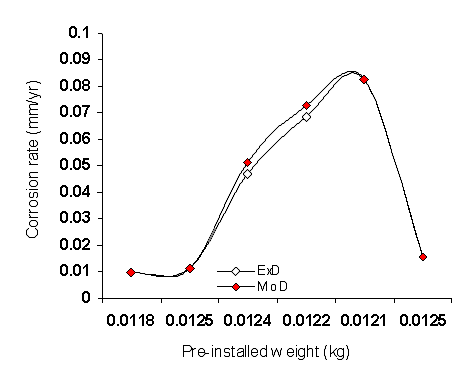• Figure 9. Comparison of the Al-Mn corrosion rate (relative to alloy pre-installed weight) as obtained from experiment and derived model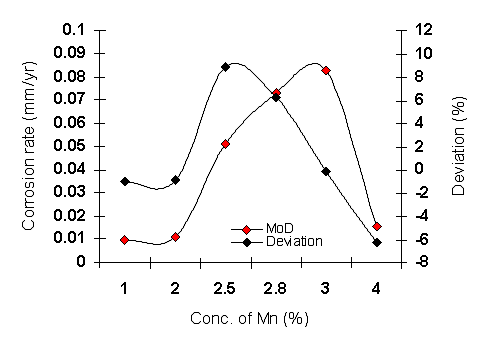• Figure 10. Variation of model-predicted corrosion rate with associated deviation from experimental results (relative to as-cast manganese content)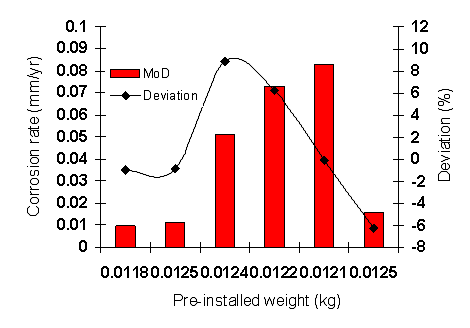• Figure 11. Variation of model-predicted corrosion rate with associated deviation from experimental results (relative to pre-installed weight)